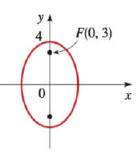Chapter 12.2, Problem 32E### Algebra and Trigonometry (MindTap ...

4th Edition
James Stewart + 2 others
ISBN: 9781305071742

#### Solutions

Chapter
Section### Algebra and Trigonometry (MindTap ...

4th Edition
James Stewart + 2 others
ISBN: 9781305071742
Textbook Problem

# 29-34 Finding the Equation of an Ellipse Find an equation for the ellipse whose graph is shown.To determine

The equation for the ellipse given in graph.

Explanation

Given:

The given graph of an ellipse is,

Figure (1)

From the graph,

Foci is,

F(0,3).

The value of a is,

b=4.

Approach:

The basic equation for an ellipse with a vertical major axis which is used is as follows,

x2b2+y2a2=1

Where a and b are constant values.

Expression to find Foci is,

F=(0,±c),

Where, c2=a2b2

Calculation:

Compare the foci of an ellipse with the standard equation of foci,

(0,±c)=(0,3)c=3

As, c2=a2b2

Substitute 3 for c and 4 for a in the above relation.

### Still sussing out bartleby?

Check out a sample textbook solution.

See a sample solution

#### The Solution to Your Study Problems

Bartleby provides explanations to thousands of textbook problems written by our experts, many with advanced degrees!

Get Started